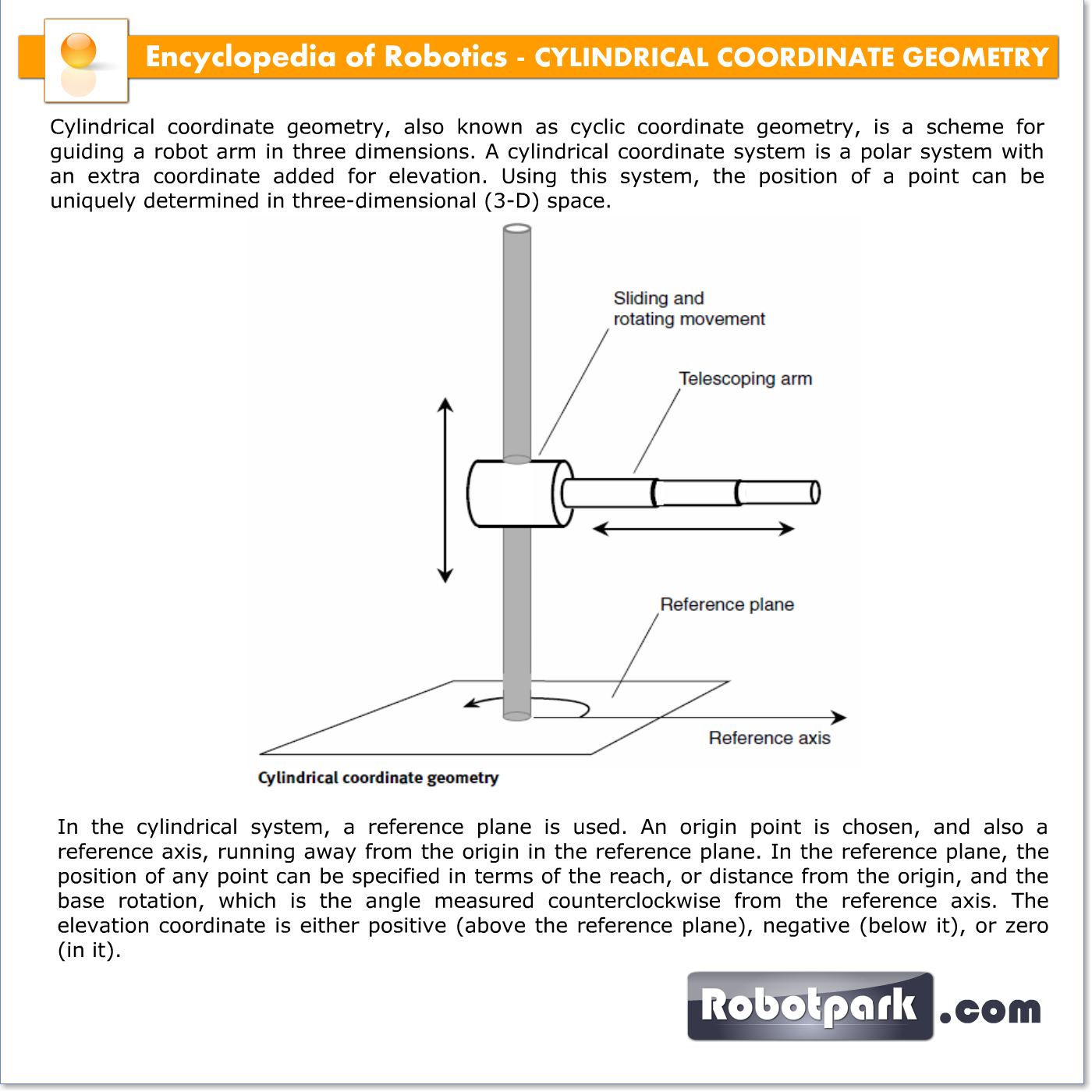# CYLINDRICAL COORDINATE GEOMETRY – 21037

Cylindrical coordinate geometry, also known as cyclic coordinate geometry, is a scheme for guiding a robot arm in three dimensions. A cylindrical coordinate system is a polar system with an extra coordinate added for elevation. Using this system, the position of a point can be uniquely determined in three-dimensional (3-D) space.In the cylindrical system, a reference plane is used. An origin point is chosen, and also a reference axis, running away from the origin in the reference plane. In the reference plane, the position of any point can be specified in terms of the reach, or distance from the origin, and the base rotation, which is the angle measured counterclockwise from the reference axis. The elevation coordinate is either positive (above the reference plane), negative (below it), or zero (in it).

The illustration shows a robot arm equipped for cylindrical coordinate geometry.